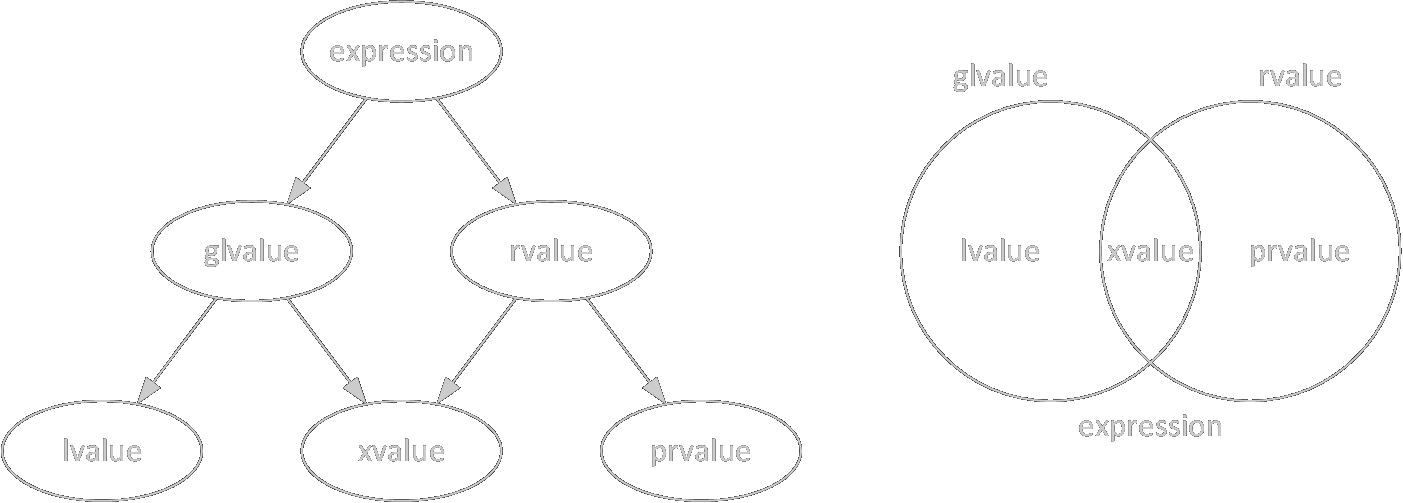# C++98/11/17表达式类别

### 目标

``````#include <utility>
#include <type_traits>

namespace cpp98
{

struct A { };
A func() { return A(); }

int main()
{
int i = 1;
i = 2;
// 3 = 4;
const int j = 5;
// j = 6;
i = j;
func() = A();
return 0;
}

}

namespace cpp11
{

#define is_lvalue(x)  std::is_lvalue_reference<decltype((x))>::value
#define is_prvalue(x) !std::is_reference<decltype((x))>::value
#define is_xvalue(x)  std::is_rvalue_reference<decltype((x))>::value
#define is_glvalue(x) (is_lvalue(x) || is_xvalue(x))
#define is_rvalue(x)  (is_xvalue(x) || is_prvalue(x))

void func();
int non_reference();
int&& rvalue_reference();
std::pair<int, int> make();

struct Test
{
int field;
void member_function()
{
static_assert(is_lvalue(field), "");
static_assert(is_prvalue(this), "");
}
enum Enum
{
ENUMERATOR,
};
};

int main()
{
int i;
int&& j = std::move(i);
Test test;

static_assert(is_lvalue(i), "");
static_assert(is_lvalue(j), "");
static_assert(std::is_rvalue_reference<decltype(j)>::value, "");
static_assert(is_lvalue(func), "");
static_assert(is_lvalue(test.field), "");
static_assert(is_lvalue("hello"), "");

static_assert(is_prvalue(2), "");
static_assert(is_prvalue(non_reference()), "");
static_assert(is_prvalue(Test{3}), "");
static_assert(is_prvalue(test.ENUMERATOR), "");

static_assert(is_xvalue(rvalue_reference()), "");
static_assert(is_xvalue(make().first), "");

return 0;
}

}

namespace reference
{

int&& rvalue_reference()
{
int local = 1;
return std::move(local);
}

const int& const_lvalue_reference(const int& arg)
{
return arg;
}

int main()
{
auto&& i = rvalue_reference();        // dangling reference
auto&& j = const_lvalue_reference(2); // dangling reference
int k = 3;
auto&& l = const_lvalue_reference(k);
return 0;
}

}

namespace auto_decl
{

int non_reference() { return 1; }
int& lvalue_reference() { static int i; return i; }
const int& const_lvalue_reference() { return lvalue_reference(); }
int&& rvalue_reference() { static int i; return std::move(i); }

int main()
{
auto [s1, s2] = std::pair(2, 3);
auto&& t1 = s1;
static_assert(!std::is_reference<decltype(s1)>::value);
static_assert(std::is_lvalue_reference<decltype(t1)>::value);

int i1 = 4;
auto i2 = i1;
decltype(auto) i3 = i1;
decltype(auto) i4{i1};
decltype(auto) i5 = (i1);
static_assert(!std::is_reference<decltype(i2)>::value, "");
static_assert(!std::is_reference<decltype(i3)>::value, "");
static_assert(!std::is_reference<decltype(i4)>::value, "");
static_assert(std::is_lvalue_reference<decltype(i5)>::value, "");

auto n1 = non_reference();
decltype(auto) n2 = non_reference();
auto&& n3 = non_reference();
static_assert(!std::is_reference<decltype(n1)>::value, "");
static_assert(!std::is_reference<decltype(n2)>::value, "");
static_assert(std::is_rvalue_reference<decltype(n3)>::value, "");

auto l1 = lvalue_reference();
decltype(auto) l2 = lvalue_reference();
auto&& l3 = lvalue_reference();
static_assert(!std::is_reference<decltype(l1)>::value, "");
static_assert(std::is_lvalue_reference<decltype(l2)>::value, "");
static_assert(std::is_lvalue_reference<decltype(l3)>::value, "");

auto c1 = const_lvalue_reference();
decltype(auto) c2 = const_lvalue_reference();
auto&& c3 = const_lvalue_reference();
static_assert(!std::is_reference<decltype(c1)>::value, "");
static_assert(std::is_lvalue_reference<decltype(c2)>::value, "");
static_assert(std::is_lvalue_reference<decltype(c3)>::value, "");

auto r1 = rvalue_reference();
decltype(auto) r2 = rvalue_reference();
auto&& r3 = rvalue_reference();
static_assert(!std::is_reference<decltype(r1)>::value, "");
static_assert(std::is_rvalue_reference<decltype(r2)>::value, "");
static_assert(std::is_rvalue_reference<decltype(r3)>::value, "");

return 0;
}

}

namespace cpp17
{

class NonMoveable
{
public:
int i = 1;
NonMoveable(int i) : i(i) { }
NonMoveable(NonMoveable&&) = delete;
};

NonMoveable make(int i)
{
return NonMoveable{i};
}

void take(NonMoveable nm)
{
return static_cast<void>(nm);
}

int main()
{
auto nm = make(2);
auto nm2 = NonMoveable{make(3)};
// take(nm);
take(make(4));
take(NonMoveable{make(5)});
return 0;
}

}

int main()
{
cpp98::main();
cpp11::main();
reference::main();
auto_decl::main();
cpp17::main();
}
``````

### C++98表达式类别

lvalue可以理解为可取地址的值，变量、对指针解引用、对返回类型为引用类型的函数的调用等，都是lvalue。临时对象都是rvalue，包括字面量和返回类型为非引用类型的函数调用等。字符串字面量是个例外，它属于不可修改的左值。

### 动机

C++11引入了右值引用和移动语义。函数返回的右值引用，顾名思义，应该表现得和右值一样，但是这会破坏很多既有的规则：

• rvalue是匿名的，不一定有存储空间，但右值引用指向内存中的具体对象，该对象还要被维护着；

• rvalue的类型是确定的，必须是完全类型，静态类型与动态类型相同，而右值引用可以是不完全类型，也可以支持多态；

• 非类类型的rvalue没有cv修饰（`const``volatile`），但右值引用可以有，而且修饰符必须保留。

• 维持右值引用是rvalue，添加一些特殊规则；

• 把右值引用归为lvalue，添加一些特殊规则；

• 细化表达式类别。

### C++11表达式类别

C++11提出了表达式类别（value category）的概念。虽然名叫“value category”，但类别划分的是表达式而不是值，所以我从标题开始就把它译为“表达式类别”。C++标准定义表达式为：

An expression is a sequence of operators and operands that specifies a computation. An expression can result in a value and can cause side effects.``````#define is_glvalue(x) (is_lvalue(x) || is_xvalue(x))
#define is_rvalue(x)  (is_xvalue(x) || is_prvalue(x))
``````

C++11对这些类别的定义如下：

• lvalue指定一个函数或一个对象；

• xvalue（eXpiring vavlue）也指向对象，通常接近其生命周期的终点；一些涉及右值引用的表达式的结果是xvalue；

• gvalue（generalized lvalue）是一个lvalue或xvalue；

• rvalue是xvalue、临时对象或它们的子对象，或者没有关联对象的值；

• prvalue（pure rvalue）是不是xvalue的rvalue。

• 变量、函数、数据成员的名字，包括右值引用类型的变量也是lvalue；

``````int i;
int&& j = std::move(i);
static_assert(is_lvalue(j), "");
static_assert(std::is_rvalue_reference<decltype(j)>::value, "");
``````
• 函数调用或重载运算符表达式，其返回类型为左值引用类型，或函数的右值引用类型；

• 内置赋值、复合赋值、前置自增、前置自减运算符表达式；

• 内置数组下标表达式`a[n]``p[n]``a`为数组类型，`p`为指针类型），`a`是一个数组lvalue；

• `a.m`，除非`m`是枚举成员，或非静态成员函数，或`a`是rvalue且`m`是非引用类型的非静态数据成员；

• `p->m`，除非`m`是枚举成员，或非静态成员函数；

• `a.*mp``a`是一个lvalue，`mp`是数据成员指针；

• `p->*mp``mp`是数据成员指针；

• 逗号表达式，第二个运算数是lvalue；

• 条件运算符`a ? b : c`，这里有非常复杂的规则，举其中一例，当`b``c`是相同类型的lvalue时；

• 字符串字面量；

• 显式转换为左值引用类型或函数的右值引用类型。

lvalue的性质：

• 与glvalue相同；

• 内置取地址运算符可以作用于lvalue；

• 可修改的lvalue可以出现在内置赋值运算符的左边；

• 可以用来初始化一个左值引用。

prvalue包括：
• 除字符串以外的字面量；

• 函数调用或重载运算符表达式，其返回类型为非引用类型；

• 内置算术运算、逻辑运算、比较运算、取地址运算符表达式；

• `a.m``p->m``m`是枚举成员或非静态成员函数（见下）；

• `a.*mp``p->*mp``mp`是成员函数指针；

• 逗号表达式，第二个运算数是rvalue；

• 条件运算符`a ? b : c`的部分情况，如`b``c`是相同类型的prvalue；

• 显式转换为非引用类型；

• `this`指针；

• 枚举成员；

• 非类型模板参数，除非它是左值引用类型；

• lambda表达式。

prvalue的性质：

• 与rvalue相同；

• 不能是多态的；

• 非类类型且非数组的prvalue没有cv修饰符，即使写了也没有；

• 必须是完全类型；

• 不能是抽象类型或其数组。

xvalue包括：
• 函数调用或重载运算符表达式，其返回类型为右值引用类型；

• 内置数组下标表达式`a[n]``a`是一个数组rvalue；

• `a.m``a`是rvalue且`m`是非引用类型的非静态数据成员；

• `a.*mp``a`是一个rvalue，`mp`是数据成员指针；

• 条件运算符`a ? b : c`的部分情况，如`b``c`是相同类型的xvalue。

xvalue的性质；

• 与rvalue相同；

• 与glvalue相同。

glvalue的性质：

• 可以隐式转换为prvalue；

• 可以是多态的；

• 可以是不完全类型。

rvalue的性质：

• 内置取地址运算符不能作用于rvalue；

• 不能出现在内置赋值或复合赋值运算符的左边；

• 可以绑定给`const`左值引用（见下）；

• 可以用来初始化右值引用（见下）；

• 如果一个函数有右值引用参数和`const`左值引用参数两个重载，传入一个rvalue时，右值引用的那个重载被调用。

• 对于非静态成员函数`mf`及其指针`pmf``a.mf``p->mf``a.*pmf``p->*pmf`都被归类为prvalue，但它们不是常规的prvalue，而是pending（即将发生的） member function call，只能用于函数调用；

• 返回`void`的函数调用、向`void`的类型装换和`throw`语句都是`void`表达式，不能用于初始化引用或函数参数；

• C++中最小的寻址单位是字节，因此位域不能绑定到非`const`左值引用上；`const`左值引用和右值引用可以绑定位域，它们指向的是位域的一个拷贝。

### 引用绑定

• 左值引用绑定lvalue，cv修饰符只能多不能少；

• 右值引用可以绑定rvalue，我们通常不给右值引用加cv修饰符；

• `const`左值引用可以绑定rvalue。

• `return`语句返回的临时对象在`return`语句结束后即销毁，这样的函数总是会返回一个空悬引用（dangling reference）；

• 绑定到初始化列表中的引用的临时对象的生命周期只延长到构造函数结束——这是个缺陷，在C++14中被修复；

• 绑定到函数参数的临时对象的生命周期延长到函数调用所在表达式结束，把该参数作为引用返回会得到空悬引用；

• 绑定到`new`表达式中的引用的临时对象的生命周期只延长到包含`new`的表达式的结束，不会跟着那个对象。

``````#include <utility>

int&& rvalue_reference()
{
int local = 1;
return std::move(local);
}

const int& const_lvalue_reference(const int& arg)
{
return arg;
}

int main()
{
auto&& i = rvalue_reference();        // dangling reference
auto&& j = const_lvalue_reference(2); // dangling reference
int k = 3;
auto&& l = const_lvalue_reference(k);
}
``````

`rvalue_reference`返回一个指向局部变量的引用，因此`i`是空悬引用；`2`绑定到`const_lvalue_reference`的参数`arg`上，函数返回后延长的生命周期达到终点，因此`j`也是悬空引用；`k`在传参的过程中根本没有临时对象创建出来，所以`l`不是空悬引用，它是指向`k``const`左值引用。

### auto与decltype

`decltype`用于声明一个类型（”declare type”），有两种语法：

• `decltype(entity)`

• `decltype(expression)`

• 如果是xvalue，产生`T&&`——`#define is_xvalue(x) std::is_rvalue_reference<decltype((x))>::value`

• 如果是lvalue，产生`T&`——`#define is_lvalue(x) std::is_lvalue_reference<decltype((x))>::value`

• 如果是prvalue，产生`T`——`#define is_prvalue(x) !std::is_reference<decltype((x))>::value`

``````#include <utility>
#include <type_traits>

int non_reference() { return 1; }
int& lvalue_reference() { static int i; return i; }
const int& const_lvalue_reference() { return lvalue_reference(); }
int&& rvalue_reference() { static int i; return std::move(i); }

int main()
{
auto [s1, s2] = std::pair(2, 3);
auto&& t1 = s1;
static_assert(!std::is_reference<decltype(s1)>::value);
static_assert(std::is_lvalue_reference<decltype(t1)>::value);

int i1 = 4;
auto i2 = i1;
decltype(auto) i3 = i1;
decltype(auto) i4{i1};
decltype(auto) i5 = (i1);
static_assert(!std::is_reference<decltype(i2)>::value);
static_assert(!std::is_reference<decltype(i3)>::value);
static_assert(!std::is_reference<decltype(i4)>::value);
static_assert(std::is_lvalue_reference<decltype(i5)>::value);

auto n1 = non_reference();
decltype(auto) n2 = non_reference();
auto&& n3 = non_reference();
static_assert(!std::is_reference<decltype(n1)>::value, "");
static_assert(!std::is_reference<decltype(n2)>::value, "");
static_assert(std::is_rvalue_reference<decltype(n3)>::value, "");

auto l1 = lvalue_reference();
decltype(auto) l2 = lvalue_reference();
auto&& l3 = lvalue_reference();
static_assert(!std::is_reference<decltype(l1)>::value, "");
static_assert(std::is_lvalue_reference<decltype(l2)>::value, "");
static_assert(std::is_lvalue_reference<decltype(l3)>::value, "");

auto c1 = const_lvalue_reference();
decltype(auto) c2 = const_lvalue_reference();
auto&& c3 = const_lvalue_reference();
static_assert(!std::is_reference<decltype(c1)>::value, "");
static_assert(std::is_lvalue_reference<decltype(c2)>::value, "");
static_assert(std::is_lvalue_reference<decltype(c3)>::value, "");

auto r1 = rvalue_reference();
decltype(auto) r2 = rvalue_reference();
auto&& r3 = rvalue_reference();
static_assert(!std::is_reference<decltype(r1)>::value, "");
static_assert(std::is_rvalue_reference<decltype(r2)>::value, "");
static_assert(std::is_rvalue_reference<decltype(r3)>::value, "");
}
``````

`auto`定义的变量都是`int`类型，无论函数的返回类型的引用和`const`修饰；用`decltype(auto)`定义的变量的类型与函数返回类型相同；`auto&&`转发引用`n3`类型为`int&&`，其余与`decltype(auto)`相同。

### C++17表达式类别

• `return`表达式中，运算数是忽略cv修饰符以后的返回类型的prvalue；

• 在初始化中，初始化器是与变量相同类型的prvalue。

• glvalue的求值能确定对象、位域、函数的身份；

• prvalue的求值初始化对象或位域，或计算运算数的值，由上下文决定；

• xvalue是表示一个对象或位域的资源能被重用的glvalue；

• lvalue是不是xvalue的glvalue；

• rvalue是prvalue或xvalue。

prvalue初始化的对象由上下文决定：在拷贝省略的情形下，prvalue不曾有关联的对象；其他情形下，prvalue将产生一个临时对象，这个过程称为临时实体化（temporary materialization）。

• 把引用绑定到prvalue上；

• 类prvalue被获取成员；

• 数组prvalue被转换为指针或下标取元素；

• prvalue出现在大括号初始化列表中，用于初始化一个`std::initializer_list<T>`

• 被使用`typeid``sizeof`运算符；

• 在语句`expr;`中或被转换成`void`，即该表达式的值被丢弃。

``````class NonMoveable
{
public:
int i = 1;
NonMoveable(int i) : i(i) { }
NonMoveable(NonMoveable&&) = delete;
};

NonMoveable make(int i)
{
return NonMoveable{i};
}

void take(NonMoveable nm)
{
return static_cast<void>(nm);
}

int main()
{
auto nm = make(2);
auto nm2 = NonMoveable{make(3)};
// take(nm);
take(make(4));
take(NonMoveable{make(5)});
}
``````

`NonMoveable`的移动构造函数被声明为`delete`，于是拷贝构造函数也被隐式`delete`。在`auto nm = make(2);`中，`NonMoveable{i}`为prvalue，根据拷贝省略的第一条规则，它直接构造为返回值；返回值是`NonMoveable`的prvalue，与`nm`类型相同，根据第二条规则，这个prvalue直接在`nm`的位置上构造；两部分结合，该声明式相当于`NonMoveable nm{2};`

### 总结

C++11规定每个表达式都属于lvalue、xvalue和prvalue三个类别之一，表达式另可分为lvalue和rvalue，或glvalue和prvalue。返回右值引用的函数调用是xvalue，右值引用类型的变量是lvalue。

`const`左值引用和右值引用可以绑定临时对象，但是临时对象的声明周期只能延长一次，返回一个指向局部变量的右值引用也会导致空悬引用。

C++17中prvalue是否有关联对象由上下文决定，拷贝省略规定了特定情况下对象不经拷贝或移动直接构造，NRVO成为强制性标准，使不能被移动的对象在语义上可以值传递。